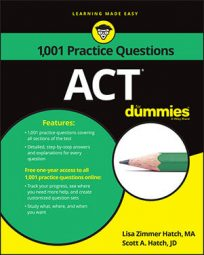##### ACTA big part of geometry involves working with angles, so it shouldn't be a surprise that the ACT Math exam contains a number of questions involving them. You may want to brush up on the properties of angles before you take on the following practice questions (and definitely before you tackle the ACT!)

## Practice questions

1. In the figure, A, B, and C are collinear.

The measure of angle ABD is 4 times that of angle DBC. What is the measure of angle ABD?

A. 36 degrees B. 45 degrees C. 72 degrees D. 108 degrees E. 144 degrees

2. The next figure shows three straight lines that intersect at point M. If angle EMF measures 47 degrees and angle AMB measures 29 degrees, what is the degree measure of angle CME?

A. 72 degrees B. 76 degrees C. 104 degrees D. 133 degrees E. 151 degrees

1. The correct answer is Choice (E).

Because the value of angle ABD measures 4 times that of angle DBC, angle ABD = 4x and angle DBC = x. Because A, B, and C are collinear, the sum of angle DBC and angle ABD is 180 degrees. To find the measure of angle ABD, set up an equation and solve for x:

Don't stop there and pick Choice (A), though. The value of x is the measure of angle DBC. Multiply 36 by 4 to get 144 degrees, which is 4x and the measure of angle ABD.

2. The correct answer is Choice (D). Scan the figure to determine which angles are equal. Angle AMB and angle DME are vertical angles, so they're equal. Angle DME also measures 29 degrees. The same goes for angle EMF and angle BMC. They're equal, so angle BMC also measures 47 degrees. The remaining two angles, angle CMD and angle AMF, are also vertical angles and equal. The degree measures of the 6 angles in the figure total to 360 degrees because they circle around the center point M. Create an equation to solve for the degree measure of the remaining two angles:

Hang on, though. This is the degree measure of angle CMD, but the question asks for the measure of angle CME. You need to add 29 degrees to 104 degrees for a degree measure of angle CME, which equals 133 degrees.